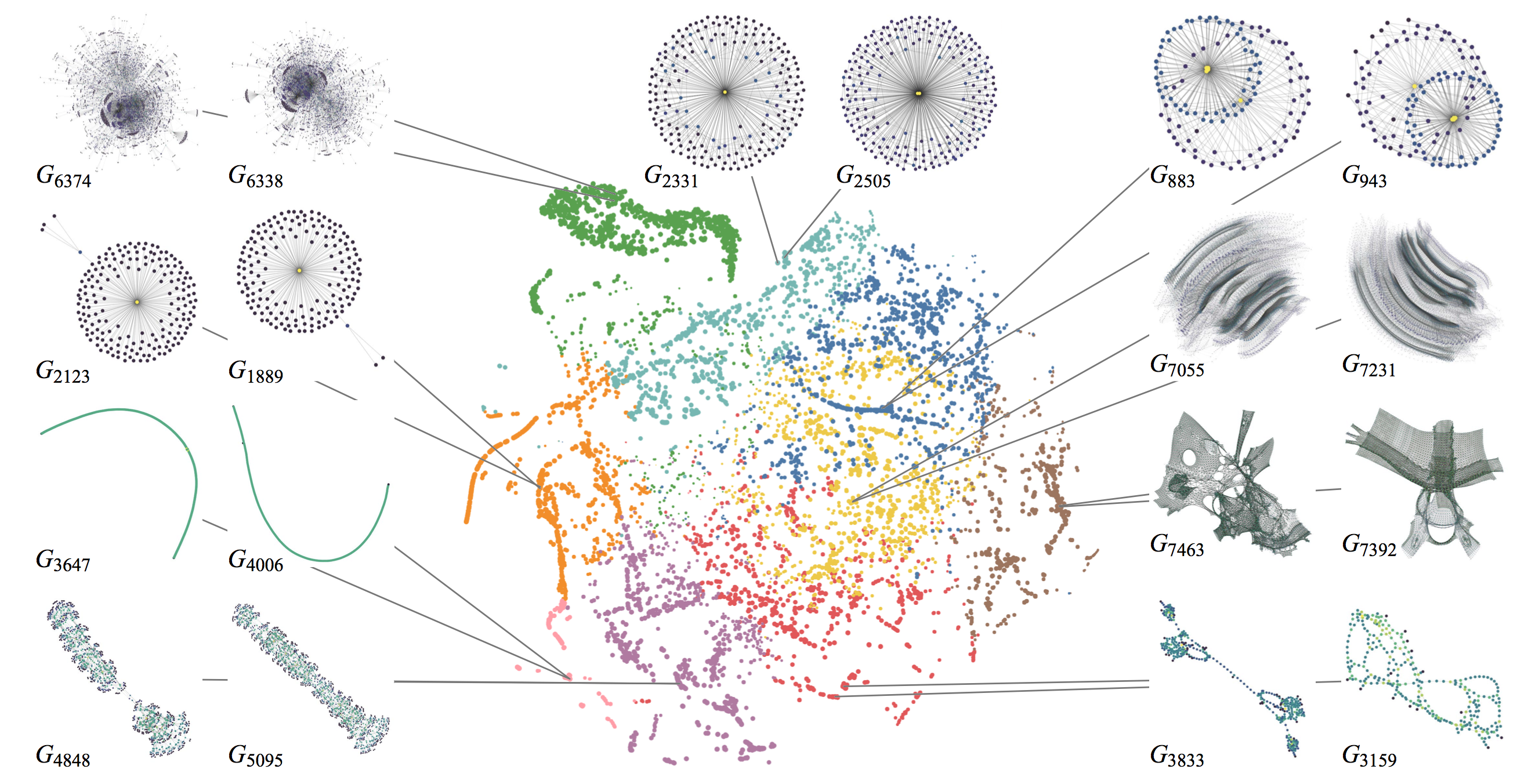• 论文原标题：What Would a Graph Look Like in This Layout? A Machine Learning Approach to Large Graph Visualization

## 个人评述

1. 特征向量只和 DGK 进行了比较，可能不够丰富

1. 本文的所有结果基于一个假设：拓扑相似的两个图，布局结果也应该相似。那么我们是否可以引申？比如图的傅里叶变换结果是否可以用于计算拓扑；如果有探索交互数据，拓扑相似的图，其探索交互过程也应该相似，就可以用来做交互推荐。

## 一、介绍

1. 一种快速、精准展示图在不同布局算法下的结果的方法；
2. 一种快速、精准的可以评估图的布局结果美学指标（aesthetic metrics）的方法；
3. 一个基于 graphlets 的设计图核的框架；
4. 一次示范，以证明图核对大规模图可视化的有效性

## 二、背景### 拓扑相似度度量

• 老方法：_isomorphsim relation, graph edit distance, graph measures_。但复杂度太高，或者普适性不强。
• 新方法图核（Graph kernel）：可以支持 kernelized machine learning，对图之间进行成对比较，得到图和图之间的拓扑相似度。可以支持 SVM 等方法。

kernel，或者叫做 kernel function，是一个比较两个输入$\chi$之间相似度的函数$\chi \times \chi \mapsto \Bbb{R}$，经常被定义为特征空间$\cal{H}$中两个向量的内积：

• _graphlets_：图元，指的是一系列异构的（_isomorphism_）导出（_indeced_）子图。

_isomophism_: two graphs $G = (V,E)$ and $G’ = (V’, E’)$ are _isomorphic_ if there exists a bijection $f: V \rightarrow V’$, called isomorphism, such that$(v_i, v_j) \in E \Leftrightarrow (f(v_i),f(v_j)) \in E’ \text{ for all }v_i,v_j \in V​$.

_indeced_: Given a graph $G$, a graph $G’ = (V’,E’)$ is a subgraph of $G$ if $V’\subseteq V$ and $E’ \subseteq E$. A subgraph $G’ = (V’,E’)$ is called an _induced_(or _vertex-induced_) subgraph of $G$ if $E’ = \{(v_i,v_j)|(v_i,v_j) \in E \text{ and }v_i, v_j \in V’\}$, that is, all edges in $E$, between two vertices $v_i,v_j \in V’$, are also present in $E’$.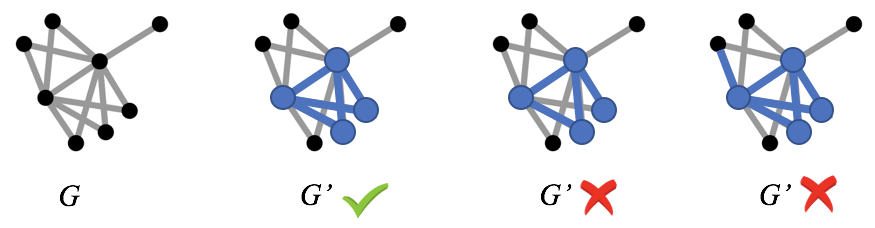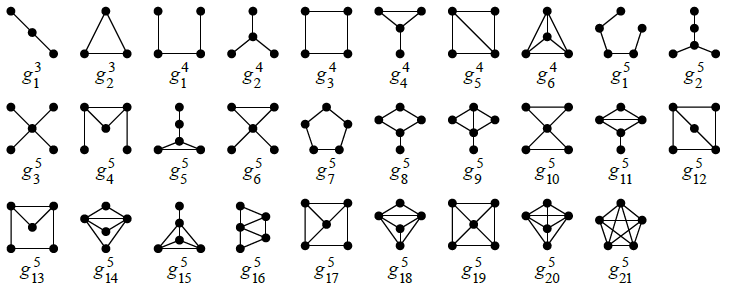$\phi$：如何对输入$x$，生成其高维特征空间$\cal H$的特征向量$\phi(x)$，以便进行内积，生成 graph kernel？这里，$x$也就是图$G$。

## 三、方法

### 设计 graphlet kernel 的框架

1. 采样图元，统计个数（采样方法）
2. 计算图元频率向量（频率计算方法）
3. 求两个图元频率向量的内积（内积方法）

#### 采样图元

• 随机节点采样（Random vertex sampling (RV)）：为了找一个 k 节点图元，通过随机找 k 个节点，用它们原有的边连接。但对于真实世界的网络，$|E|\ll O(|V|^2)$，那么大部分随机采样的图元将会是不连通的。当只想采样连通图元的话，就需要很多轮迭代才能采到足够数量的图元，时间开销会很大。
• 随机游走采样（Random walk sampling (RW)）：基于随机游走的采样方法，还没被用于设计 graphlet kernel，这种采样方法可以用来采集连通图元。

#### 计算图元频率向量

• 线性比例变换（linear scale, LIN）：也被叫做图元浓度（graphlet concentration），是每种图元在图中的百分比，一些工作用每种图元的加权数量$w_i$来定义它（文中并未指定权重$w_i$的定义）：

• 对数比例变换（Logarithmic scale, LOG）：类似于节点度分布，图元频率分布也经常服从幂律分布，这会导致缺少关键信息的图元会远高于那些信息丰富的图元，故而一些工作用了一个对数变换来解决这个问题，其中$w_b$是一个基础权重，来防止$log(0)$:

#### 定义内积

• 余弦相似度（Cosine similarity，COS）：现有最多的 graphlet kernel 使用欧氏空间中的两个向量的点积后产生的点积产生的矩阵的归一化结果作为核函数，也就是两个向量的余弦相似度，也是两个向量的

• 高斯径向基函数核（Gaussian radial basis function kernel, RBF）：经常被用在机器学习中，$\sigma$是一个自由参数

• 拉普拉斯核（Laplacian kernel，LAPLACIAN）：是 RBF 核的一种变种：

### 预测图的布局结果（WGL）

1. 计算准备好的已存在的图（每个图都预先计算好了布局结果）和输入图$G_{input}$的相似度
2. 移除不满足约束条件的图（因为有些图，虽然它的特征向量和$G_{input}$很相似，但是因为节点过多或者过少，事实上应该不算做相似图，比如下图：图元频率分布的四个例子，文章中设置了一个约束条件，即相似图的节点数，不能多于$G_{input}$的 2 倍，或者少于$G_{input}$的 1/2）
3. 选出$k$个最相似图
###### 图元频率分布的四个例子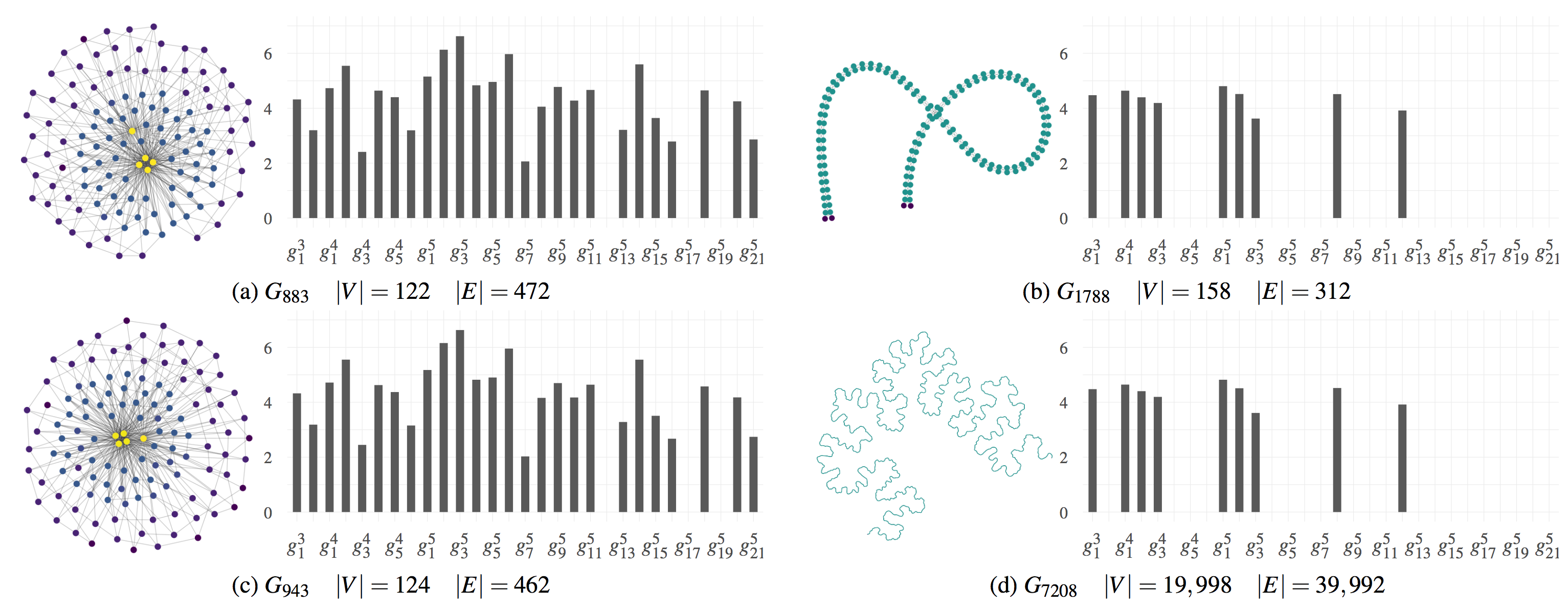### 预测图的美学指标（EAM）

1. 准备训练数据（一系列的图，以及它们在不同布局下的布局结果和相关的美学指标）
2. 用 graph kernel 计算训练数据中的图和图之间的相似度
3. 训练回归模型

## 四、评估

### 估算布局的美学指标

#### 评估目的

1. 精确？在不计算实际布局的情况下，是否能够精确地估算布局的美学指标？
2. 快速？能否快速的获得估算结果？
3. 更强？由上述框架产生的 graph kernel 是否能够在计算时间和精度方面都优于最先进的 graph kernel？

#### 实验设计

##### 数据集
1. 收集了包括并不限于社交网络、网络文档网络、几何网络的 3700 个图。在不失一般性的情况下，把具有多个连通部件的图拆成几个分离的图（一个连通部件对应一个图）。
2. 之后剔除少于 100 个节点的图，因为这些图的计算会特别快，从而影响实验结果。
3. 最后剩下 8263 个图，节点数范围从 100~一亿一千万，边数范围从 100~18 亿。因为某些图在计算布局的时间上花了 10 天以上或者内存跑完了，这些图就被抛弃了。

##### 布局方法选取

• 力引导（Force-directed methods）：电子模型中选取了 Fruchterman-Reingold (FR)，能量模型中选取了 Kamada-Kawai (KK)
• 基于降维的方法（Dimension reduction based method）：选取了 High-Dimensional Embedder (HDE)
• 谱方法（Spectral method）：选取了 Koren 的方法
• 多层方法（Multi-Level methods）：选了 sfdp 和 FM3
• 基于聚类的方法（Clustering based methods）：选了 Treemap based layout 和 the Gosper curve based layout
##### 美学指标的选取

• Crosslessness ($m_c$)：在很多研究中，边的交叉数量被作为一种最重要的审美标准，边交叉越少越好：

其中，$c$是边的交叉数，$c_{max}$是边交叉数的近似上界，被定义为：

• Minimum angle metric ($m_a$)：被定义为点$v$上的边的最小夹角和理想的最小夹角的平均绝对离差：

其中，理想最小夹角，定义为点上的所有边刚好平分 360°：

• Edge length variation ($m_l$ )：根据边长的变异系数计算得到：

其中:

• $l_{cv}$是边长的变异系数
• $l_\sigma$是边长的标准差
• $l_\mu$是边长的平均值
• Shape-based metric($m_s$)：可以用 mean Jaccard similarity(MJS)方法，比较某个图和标准图（一般认为标准图的结构良好，属于较美的图）的相似度，定义该图的$m_s$

其中，$G_1=(V, E_1)$和$G_2 = (V, E_2)$是所有节点都相同的两个图，$N_i(v)$是图$G_i$中$v$节点的相邻节点。采用Gabriel graph作为标准图，用于比较。

#### 如何评判估算结果是否精确

• 均方根误差（Root-Mean-Square Error (RMSE)），结果越小越好

其中：

• $\cal Y = \{y_1,…,y_n\}$是经过实际布局后，测量出来的真实结果；
• $\tilde{\cal Y} = \{\tilde{y_1},…,\tilde{y_n}\}$是回归模型的估算结果。
• 测定系数（coefficient of determination (R ) ）：结果越小越好

其中$y_\mu$是测量结果$y_i$的平均值

#### 实验结果

##### 估算精度方面
###### 部分精度评估结果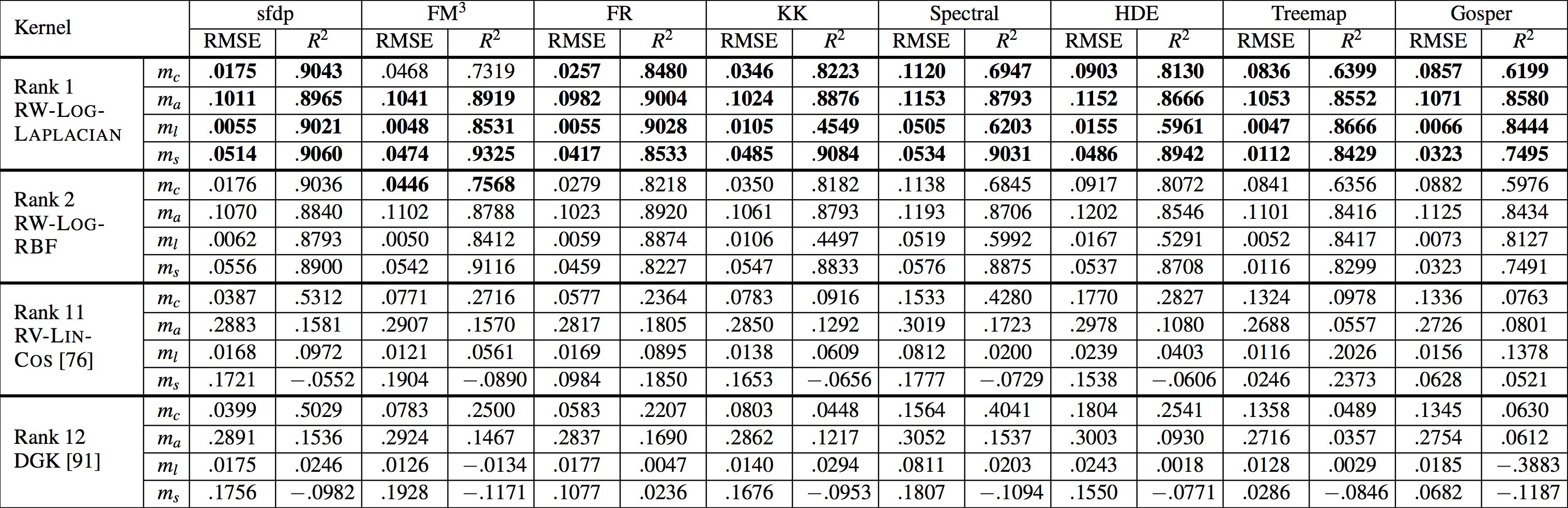• 从上图可以看到，除了在$FM^3$布局下的$m_c$指标以外，RW-LOG-LAPLACIAN kernel 的表现都是最佳。而 RW-LOG-RBF kernel 则在$FM^3$布局下的$m_c$指标表现最佳。DGK 排名为第十二名，说明框架产生的 kernel 大部分都优于这个对照组，也就回答了第三个问题。
• 总体而言，RW 采样方法得到的结果精确度要高于 RV 采样方法，LOG 变换比 LIN 变化的精度更高，用 LAPLACIAN 的核也比其他两者精度更高。
##### 计算时间方面

• RW 采样计算速度最快，每幅图平均 0.14089 秒 (标准差为 1.9559)。
• DGK 的图元采样速度比 RW 要慢，每幅图平均 3.38 秒（标准差为 7.88）。
• RV 采样计算速度最慢，每幅图平均 6.81 秒（标准差为 7.04）。
###### 计算时间和图规模之间的关系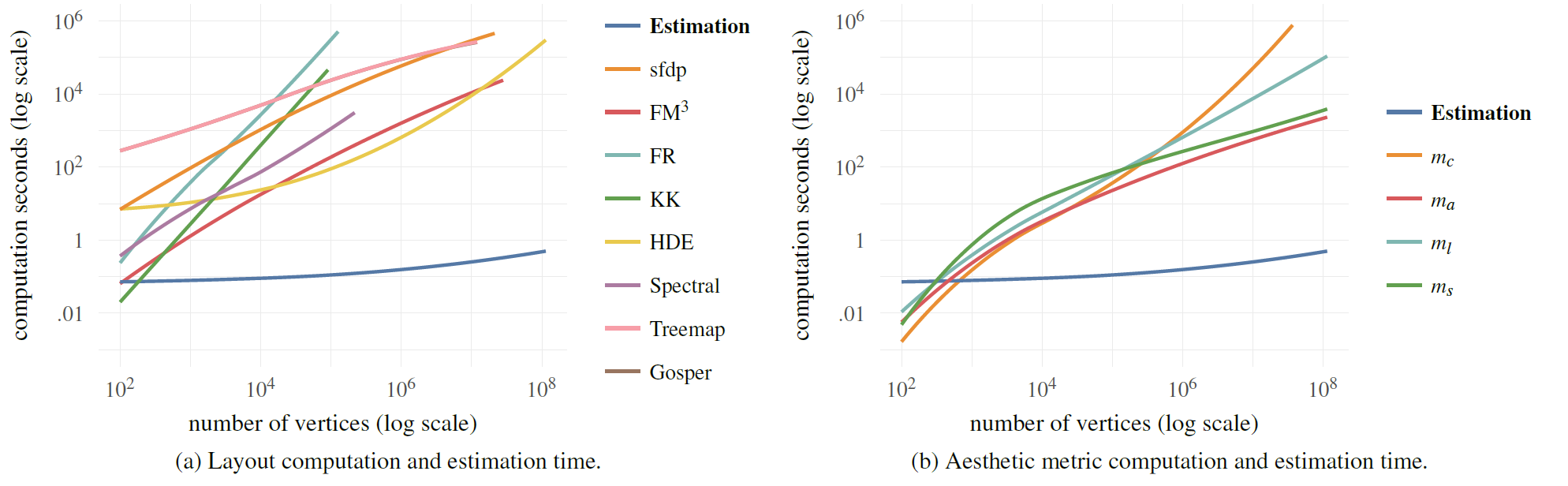#### 讨论

• RW 采样方法比 RV 采样方法精度高，因为 RW 只采样连通图元，故而我们推测，连通图元对展现图的结构信息更重要，故而估算结果的精度也更高。
• 事实上，在用 RV 采样的过程中，每个图采样 10000 个图元，发现只有 1.913%是连通图元，而有 35.77%的图没有采样到连通图元，即便它们这些图本身都是连通的，这就使得这些图本身就很难用于比较。
• 使用 LOG 变换的核，比使用 LIN 变换的核，平均而言有着更高的估算精度，可能是因为图元的分布也经常呈现出幂率分布。
• 因为在估算过程中，不用再计算实际的布局结果，所以估算布局结果和美学指标的速度会很快。
• 发现除了 DGK 以外，其他核矩阵的计算时间都很短，而且相差不大，平均 5.99 秒，标准差 3.26。而 DGK 因为要计算语言模型 language modeling，需要平均 182.96 秒。

### 预测图的布局结果

#### 实验设计

##### 任务

###### 用户调研中的其中一个任务示例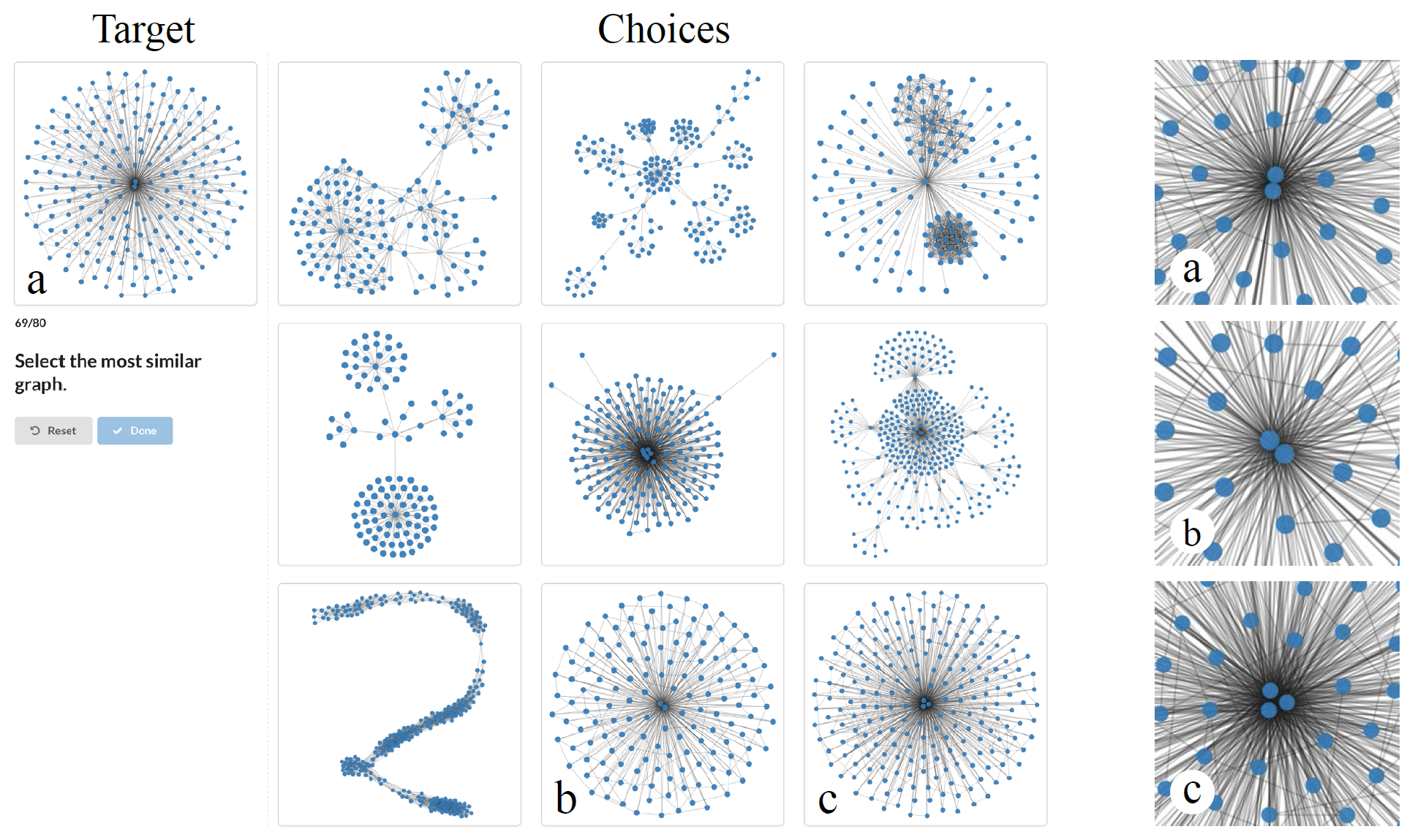##### 图的选取
• 目标图（Target）：一共选取了 9 个目标图，选择过程如下：
1. 将已有的 8000+图，利用谱聚类（spectral clustering）的方法分成 9 类
2. 对于每一类，选出相互之间拓扑相似度最高的十个图，作为这一类的代表图
3. 从这十个代表图中随机挑选一个作为目标图（Target)
• 选项图（Choice）：每幅目标图，都要挑选九幅选项图：
1. 利用每幅图和目标图之间的相似度，利用Jenks natuarak breaks 方法（一种一维的 K 均值分类方法）将所有图分成 9 类
2. 从与目标图最相似的聚类中，选出和目标图相似度最高的图，作为其中一张选项图
3. 剩余八类中，每一类都选出相互之间拓扑相似度最高的十个图，再从这十张图中随机挑选一张，作为剩余八张选项图
4. 当然在挑选过程中，需要过滤掉那些节点数大于目标图两倍，或者小于目标图一半的图

#### 实验结果

###### 用户调研的总结• 如图：用户调研的总结，发现有 80.46%的情况，参与者认为最相似的选项图（$r_P=1$）和系统选出来的最相似的图（$r_T=1$）刚好吻合（图 a 最左边的蓝色长条）；90.27%的情况下，参与者认为的最相似（$r_P=1$）和第二相似（$r_P=2$）的选项图和系统选出的最相似图（$r_T=1$）吻合；而 93.8%的情况下，参与者选出的第 1、2、3 相似的图（$r_P={1,2,3}$）和系统选出的最相似图（$r_T=1$）吻合。
• 除了 treemap 布局和 spectral 布局以外，有 78.52%~93.33%的情况下，参与者选出来的最相似图和算法得到的最相似图一致，有 94.44%~99.26%的情况下，参与者选出来的最相似的三幅图和算法得到的最相似图吻合。
• 目标图中，除了$G_{2331}$和$G_{3833}$，有 79.58%~98.33%的情况下，参与者选出来的最相似图和算法得到的最相似图一致，有 92.08%~99.99%的情况下，参与者选出来的最相似的三幅图和算法得到的最相似图吻合。

#### 讨论

• 为什么 Spectral 和 Treemap 布局比其他的布局方法结果要差？
• spectral：参与者根据线的形状、节点的个数来判断相似程度，我们发现，谱布局，经常会有很多节点重叠，故而会让参与者判断不准
• treemap：因为它的几何限制，经常产生相似的图形，参与者几乎都提到，“这些图怎么长的都一样？”，他们经常会根据边的密度，整体边的走向来判断相似程度，故而判断不准
• 为什么 G2331 情况比较糟糕？图 5所示情况，参与者在 b 和 c 图中徘徊不定：
• 参与者选择 c 的原因：密度、形状、边的数量
• 参与者选择 b 的原因：中心节点的数量
• 系统选择 c 的原因：大体上结构和目标相似

## 五、讨论